Publicité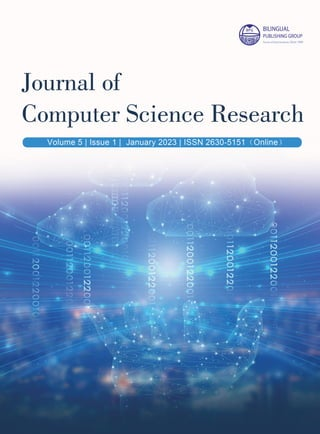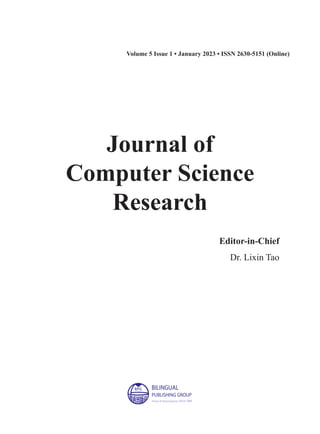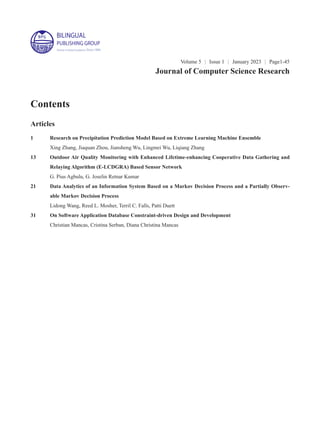Publicité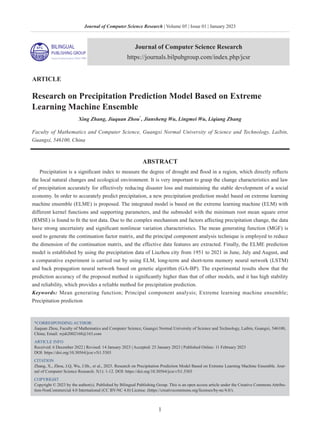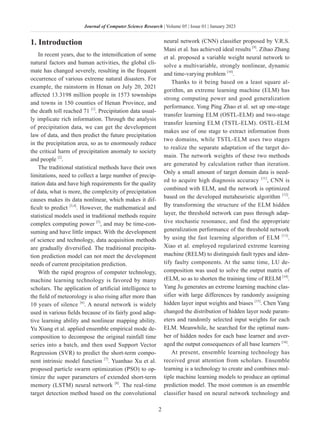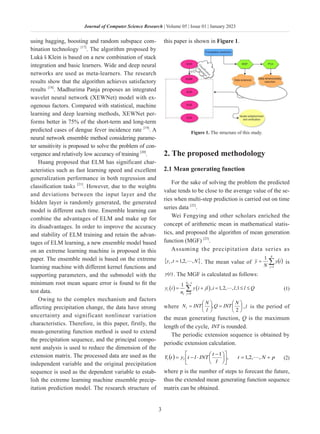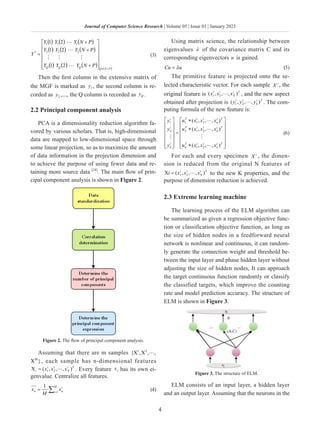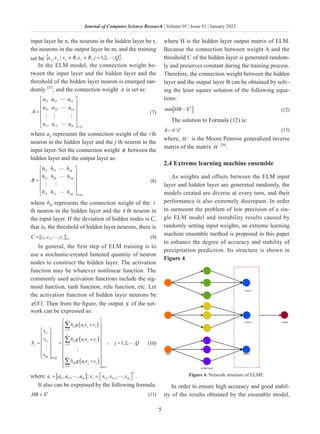Publicité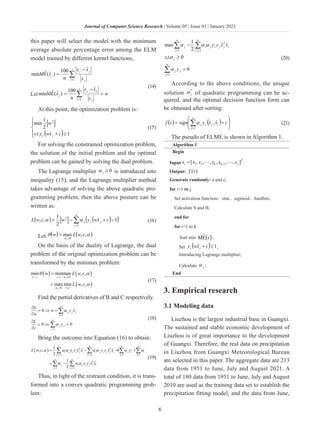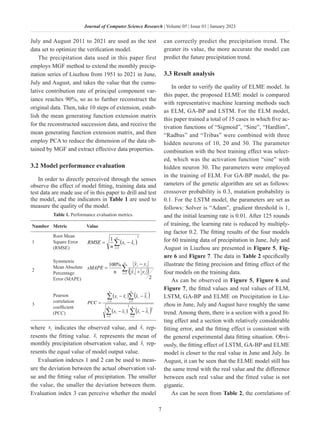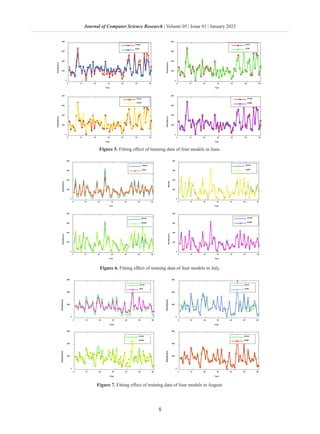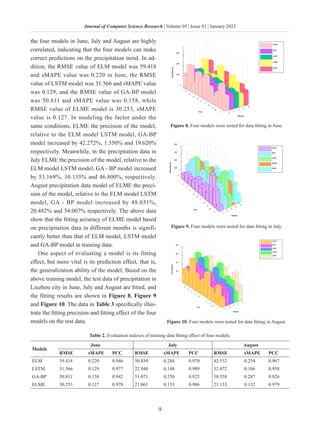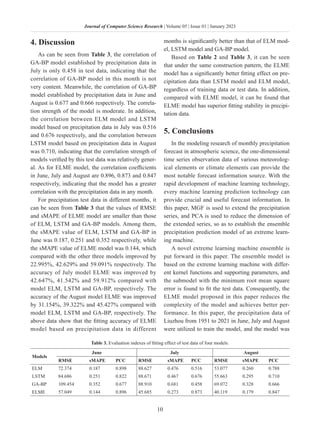Publicité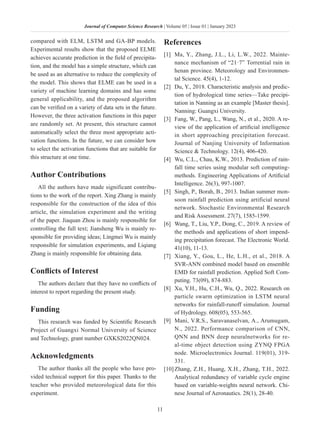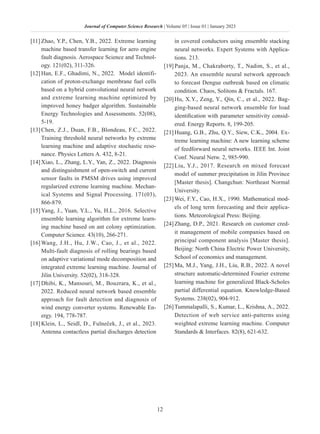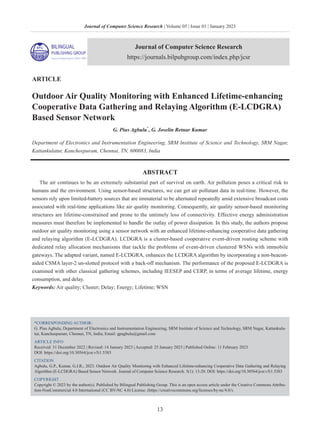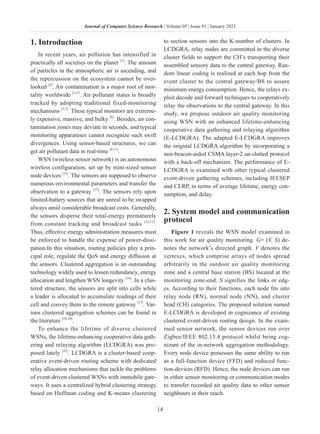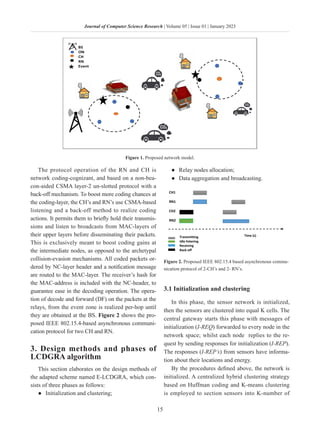Publicité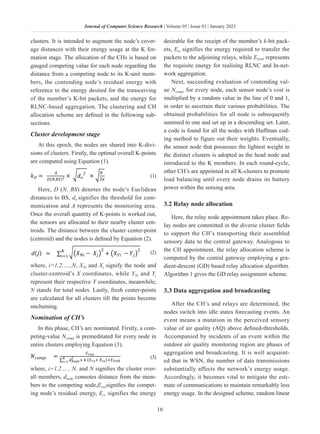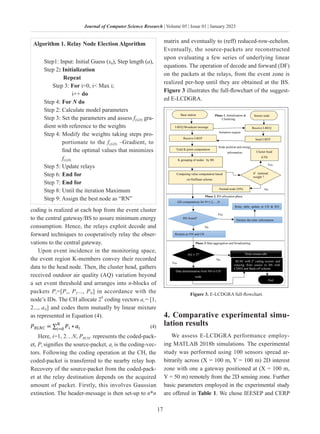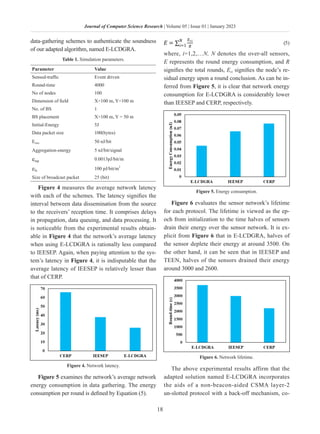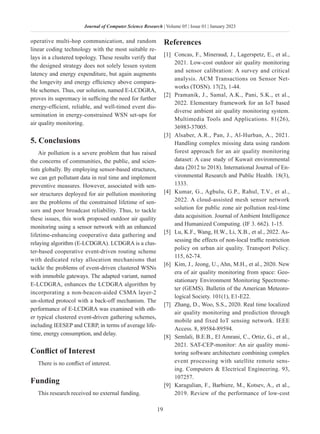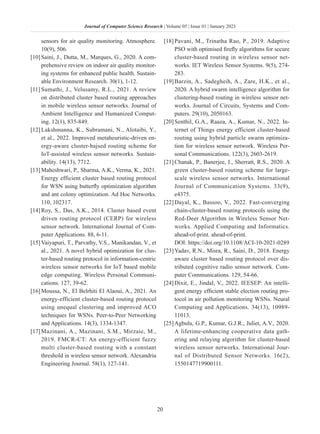Publicité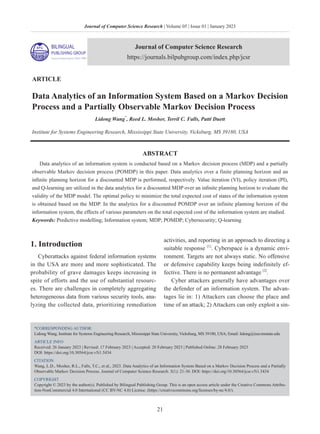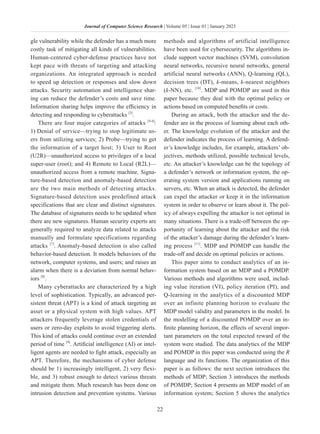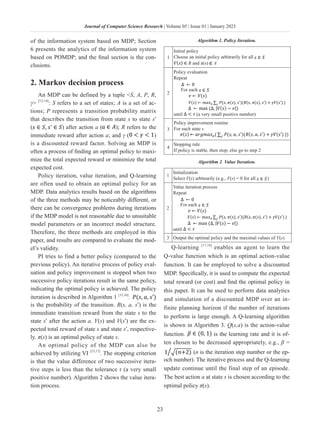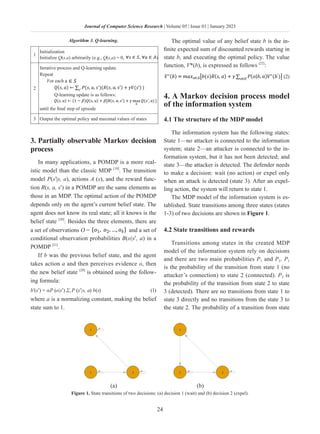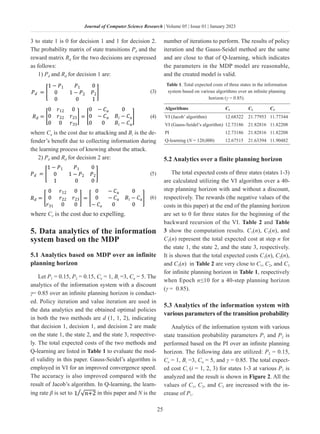Publicité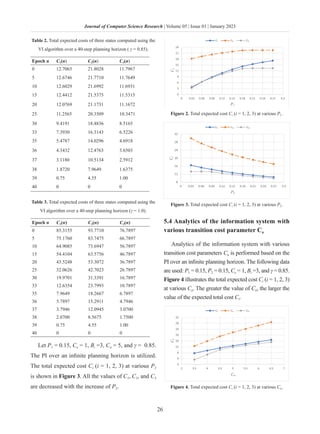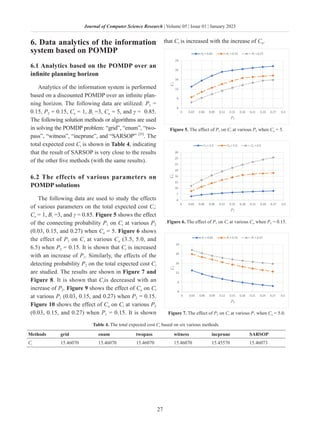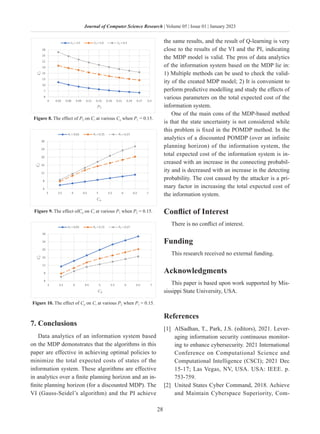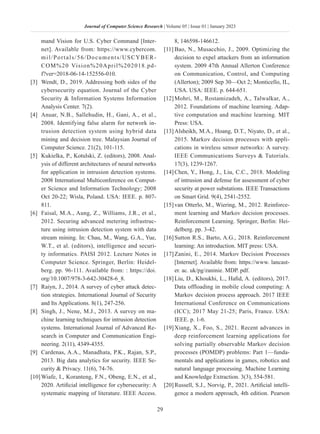Publicité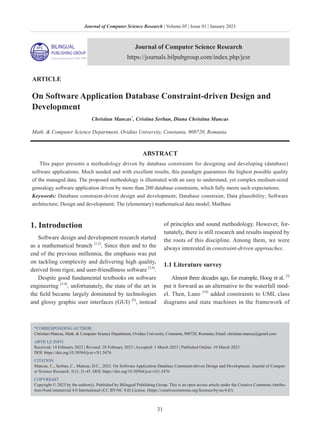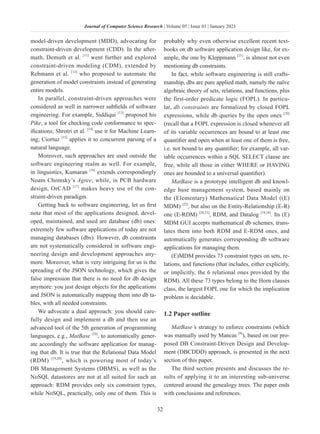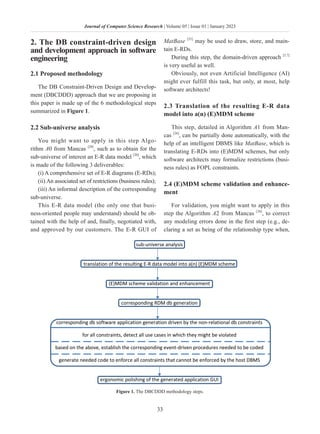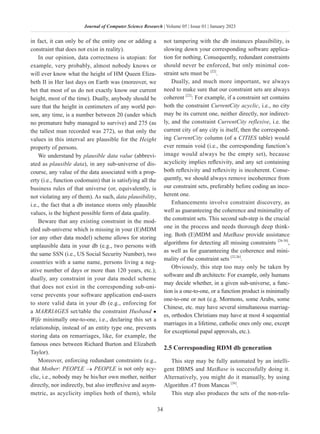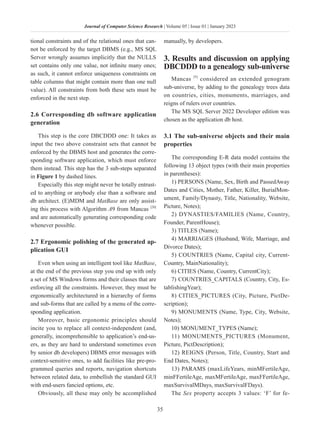Publicité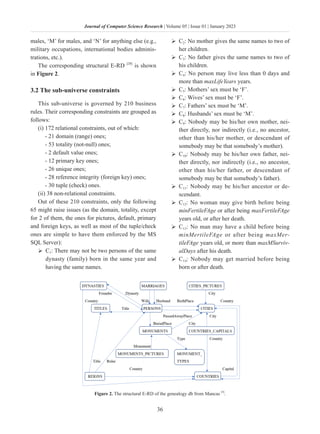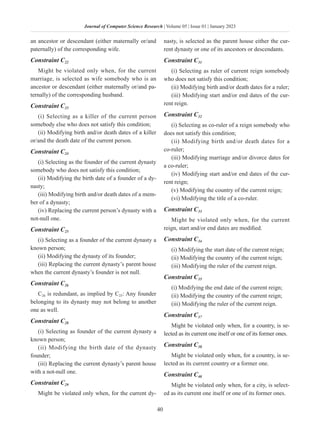Publicité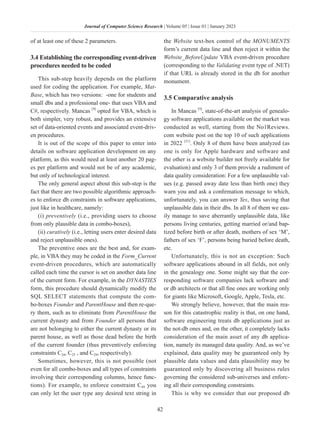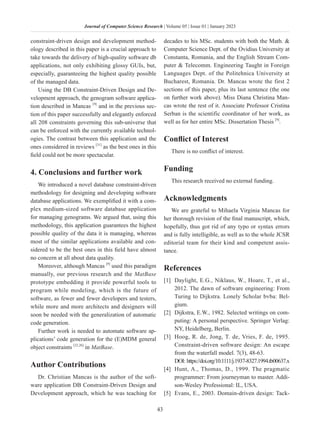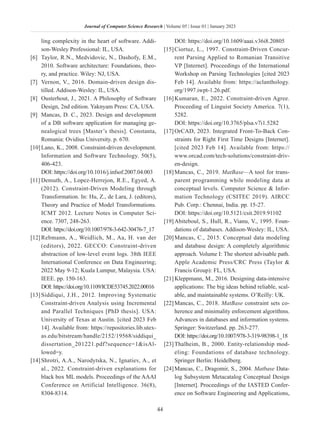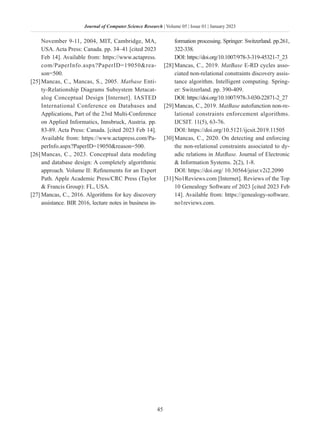Prochain SlideShare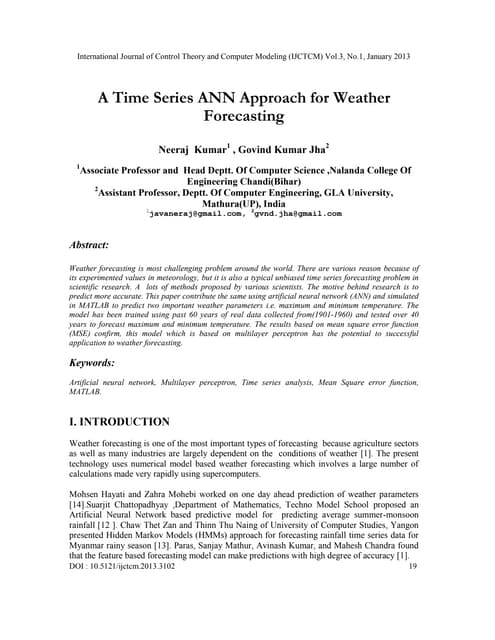A Time Series ANN Approach for Weather Forecasting
Chargement dans ... 3
1 sur 50
Publicité

### Journal of Computer Science Research | Vol.5, Iss.1 January 2023

1. Editor-in-Chief Dr. Lixin Tao Editorial Board Members Pace University, United States Yuan Liang, China Chunqing Li, China Roshan Chitrakar, Nepal Omar Abed Elkareem Abu Arqub, Jordan Lian Li, China Zhanar Akhmetova, Kazakhstan Hashiroh Hussain, Malaysia Imran Memon, China Aylin Alin, Turkey Xiqiang Zheng, United States Manoj Kumar, India Awanis Romli, Malaysia Manuel Jose Cabral dos Santos Reis, Portugal Zeljen Trpovski, Serbia Degan Zhang, China Shijie Jia, China Marbe Benioug, China Kamal Ali Alezabi, Malaysia Xiaokan Wang, China Rodney Alexander, United States Hla Myo Tun, Myanmar Nur Sukinah Aziz, Malaysia Shumao Ou, United Kingdom Serpil Gumustekin Aydin, Turkey Nitesh Kumar Jangid, India Xiaofeng Yuan, China Michalis Pavlidis, United Kingdom Dileep M R, India Jie Xu, China Qian Yu, Canada Jerry Chun-Wei Lin, Norway Paula Maria Escudeiro, Portugal Mustafa Cagatay Korkmaz, Turkey Mingjian Cui, United States Besir Dandil, Turkey Jose Miguel Canino-Rodríguez, Spain Lisitsyna Liubov, Russian Federation Chen-Yuan Kuo, United States Antonio Jesus Munoz Gallego, Spain Ting-Hua Yi, China Norfadilah Kamaruddin, Malaysia Lanhua Zhang, China Samer Al-khateeb, United States Neha Verma, India Viktor Manahov, United Kingdom Gamze Ozel Kadilar, Turkey Ebba S I Ossiannilsson, Sweden Aminu Bello Usman, United Kingdom Vijayakumar Varadarajan, Australia Patrick Dela Corte Cerna, Ethiopia Dariusz Jacek Jakóbczak, Poland
2. Volume 5 Issue 1 • January 2023 • ISSN 2630-5151 (Online) Journal of Computer Science Research Editor-in-Chief Dr. Lixin Tao
3. Volume 5 ｜ Issue 1 ｜ January 2023 ｜ Page1-45 Journal of Computer Science Research Contents Articles 1 Research on Precipitation Prediction Model Based on Extreme Learning Machine Ensemble Xing Zhang, Jiaquan Zhou, Jiansheng Wu, Lingmei Wu, Liqiang Zhang 13 Outdoor Air Quality Monitoring with Enhanced Lifetime-enhancing Cooperative Data Gathering and Relaying Algorithm (E-LCDGRA) Based Sensor Network G. Pius Agbulu, G. Joselin Retnar Kumar 21 Data Analytics of an Information System Based on a Markov Decision Process and a Partially Observ- able Markov Decision Process Lidong Wang, Reed L. Mosher, Terril C. Falls, Patti Duett 31 On Software Application Database Constraint-driven Design and Development Christian Mancas, Cristina Serban, Diana Christina Mancas
4. 1 Journal of Computer Science Research | Volume 05 | Issue 01 | January 2023 Journal of Computer Science Research https://journals.bilpubgroup.com/index.php/jcsr *CORRESPONDING AUTHOR: Jiaquan Zhou, Faculty of Mathematics and Computer Science, Guangxi Normal University of Science and Technology, Laibin, Guangxi, 546100, China; Email: wjsh2002168@163.com ARTICLE INFO Received: 6 December 2022 | Revised: 14 January 2023 | Accepted: 25 January 2023 | Published Online: 11 February 2023 DOI: https://doi.org/10.30564/jcsr.v5i1.5303 CITATION Zhang, X., Zhou, J.Q, Wu, J.Sh., et al., 2023. Research on Precipitation Prediction Model Based on Extreme Learning Machine Ensemble. Jour- nal of Computer Science Research. 5(1): 1-12. DOI: https://doi.org/10.30564/jcsr.v5i1.5303 COPYRIGHT Copyright © 2023 by the author(s). Published by Bilingual Publishing Group. This is an open access article under the Creative Commons Attribu- tion-NonCommercial 4.0 International (CC BY-NC 4.0) License. (https://creativecommons.org/licenses/by-nc/4.0/). ARTICLE Research on Precipitation Prediction Model Based on Extreme Learning Machine Ensemble Xing Zhang, Jiaquan Zhou* , Jiansheng Wu, Lingmei Wu, Liqiang Zhang Faculty of Mathematics and Computer Science, Guangxi Normal University of Science and Technology, Laibin, Guangxi, 546100, China ABSTRACT Precipitation is a significant index to measure the degree of drought and flood in a region, which directly reflects the local natural changes and ecological environment. It is very important to grasp the change characteristics and law of precipitation accurately for effectively reducing disaster loss and maintaining the stable development of a social economy. In order to accurately predict precipitation, a new precipitation prediction model based on extreme learning machine ensemble (ELME) is proposed. The integrated model is based on the extreme learning machine (ELM) with different kernel functions and supporting parameters, and the submodel with the minimum root mean square error (RMSE) is found to fit the test data. Due to the complex mechanism and factors affecting precipitation change, the data have strong uncertainty and significant nonlinear variation characteristics. The mean generating function (MGF) is used to generate the continuation factor matrix, and the principal component analysis technique is employed to reduce the dimension of the continuation matrix, and the effective data features are extracted. Finally, the ELME prediction model is established by using the precipitation data of Liuzhou city from 1951 to 2021 in June, July and August, and a comparative experiment is carried out by using ELM, long-term and short-term memory neural network (LSTM) and back propagation neural network based on genetic algorithm (GA-BP). The experimental results show that the prediction accuracy of the proposed method is significantly higher than that of other models, and it has high stability and reliability, which provides a reliable method for precipitation prediction. Keywords: Mean generating function; Principal component analysis; Extreme learning machine ensemble; Precipitation prediction
5. 2 Journal of Computer Science Research | Volume 05 | Issue 01 | January 2023 1. Introduction In recent years, due to the intensification of some natural factors and human activities, the global cli- mate has changed severely, resulting in the frequent occurrence of various extreme natural disasters. For example, the rainstorm in Henan on July 20, 2021 affected 13.3198 million people in 1573 townships and towns in 150 counties of Henan Province, and the death toll reached 71  . Precipitation data usual- ly implicate rich information. Through the analysis of precipitation data, we can get the development law of data, and then predict the future precipitation in the precipitation area, so as to enormously reduce the critical harm of precipitation anomaly to society and people  . The traditional statistical methods have their own limitations, need to collect a large number of precip- itation data and have high requirements for the quality of data, what is more, the complexity of precipitation causes makes its data nonlinear, which makes it dif- ficult to predict [3,4] . However, the mathematical and statistical models used in traditional methods require complex computing power  , and may be time-con- suming and have little impact. With the development of science and technology, data acquisition methods are gradually diversified. The traditional precipita- tion prediction model can not meet the development needs of current precipitation prediction. With the rapid progress of computer technology, machine learning technology is favored by many scholars. The application of artificial intelligence to the field of meteorology is also rising after more than 10 years of silence  . A neural network is widely used in various fields because of its fairly good adap- tive learning ability and nonlinear mapping ability. Yu Xiang et al. applied ensemble empirical mode de- composition to decompose the original rainfall time series into a batch, and then used Support Vector Regression (SVR) to predict the short-term compo- nent intrinsic model function  . Yuanhao Xu et al. proposed particle swarm optimization (PSO) to op- timize the super parameters of extended short-term memory (LSTM) neural network  . The real-time target detection method based on the convolutional neural network (CNN) classifier proposed by V.R.S. Mani et al. has achieved ideal results  . Zihao Zhang et al. proposed a variable weight neural network to solve a multivariable, strongly nonlinear, dynamic and time-varying problem  . Thanks to it being based on a least square al- gorithm, an extreme learning machine (ELM) has strong computing power and good generalization performance. Yong Ping Zhao et al. set up one-stage transfer learning ELM (OSTL-ELM) and two-stage transfer learning ELM (TSTL-ELM). OSTL-ELM makes use of one stage to extract information from two domains, while TSTL-ELM uses two stages to realize the separate adaptation of the target do- main. The network weights of these two methods are generated by calculation rather than iteration. Only a small amount of target domain data is need- ed to acquire high diagnosis accuracy  , CNN is combined with ELM, and the network is optimized based on the developed metaheuristic algorithm  . By transforming the structure of the ELM hidden layer, the threshold network can pass through adap- tive stochastic resonance, and find the appropriate generalization performance of the threshold network by using the fast learning algorithm of ELM  . Xiao et al. employed regularized extreme learning machine (RELM) to distinguish fault types and iden- tify faulty components. At the same time, LU de- composition was used to solve the output matrix of rELM, so as to shorten the training time of RELM  . Yang Ju generates an extreme learning machine clas- sifier with large differences by randomly assigning hidden layer input weights and biases  . Chen Yang changed the distribution of hidden layer node param- eters and randomly selected input weights for each ELM. Meanwhile, he searched for the optimal num- ber of hidden nodes for each base learner and aver- aged the output consequences of all base learners  . At present, ensemble learning technology has received great attention from scholars. Ensemble learning is a technology to create and combines mul- tiple machine learning models to produce an optimal prediction model. The most common is an ensemble classifier based on neural network technology and
6. 3 Journal of Computer Science Research | Volume 05 | Issue 01 | January 2023 using bagging, boosting and random subspace com- bination technology  . The algorithm proposed by Luká š Klein is based on a new combination of stack integration and basic learners. Wide and deep neural networks are used as meta-learners. The research results show that the algorithm achieves satisfactory results  . Madhurima Panja proposes an integrated wavelet neural network (XEWNet) model with ex- ogenous factors. Compared with statistical, machine learning and deep learning methods, XEWNet per- forms better in 75% of the short-term and long-term predicted cases of dengue fever incidence rate  . A neural network ensemble method considering parame- ter sensitivity is proposed to solve the problem of con- vergence and relatively low accuracy of training  . Huang proposed that ELM has significant char- acteristics such as fast learning speed and excellent generalization performance in both regression and classification tasks  . However, due to the weights and deviations between the input layer and the hidden layer is randomly generated, the generated model is different each time. Ensemble learning can combine the advantages of ELM and make up for its disadvantages. In order to improve the accuracy and stability of ELM training and retain the advan- tages of ELM learning, a new ensemble model based on an extreme learning machine is proposed in this paper. The ensemble model is based on the extreme learning machine with different kernel functions and supporting parameters, and the submodel with the minimum root mean square error is found to fit the test data. Owing to the complex mechanism and factors affecting precipitation change, the data have strong uncertainty and significant nonlinear variation characteristics. Therefore, in this paper, firstly, the mean-generating function method is used to extend the precipitation sequence, and the principal compo- nent analysis is used to reduce the dimension of the extension matrix. The processed data are used as the independent variable and the original precipitation sequence is used as the dependent variable to estab- lish the extreme learning machine ensemble precip- itation prediction model. The research structure of this paper is shown in Figure 1. Figure 1. The structure of this study. 2. The proposed methodology 2.1 Mean generating function For the sake of solving the problem the predicted value tends to be close to the average value of the se- ries when multi-step prediction is carried out on time series data  . Wei Fengying and other scholars enriched the concept of arithmetic mean in mathematical statis- tics, and proposed the algorithm of mean generation function (MGF)  . Assuming the precipitation data series as { } N t yt , , 2 , 1 ,  = . The mean value of ( ) ∑ = = N j i y N y 1 1 is ) (t y . The MGF is calculated as follows: ( ) ( ) 1 0 1 , 1,2, , ,1 l N l j l y i y i jl i l l Q n − = = + = ≤ ≤ ∑  (1) where       =       = 2 , N INT Q l N INT Nl ,l is the period of the mean generating function, Q is the maximum length of the cycle, INT is rounded. The periodic extension sequence is obtained by periodic extension calculation. ( ) p N t l t INT l t y t Y l l + =             − ⋅ − = , , 2 , 1 , 1  (2) where p is the number of steps to forecast the future, thus the extended mean generating function sequence matrix can be obtained.
7. 4 Journal of Computer Science Research | Volume 05 | Issue 01 | January 2023 ( ) ( ) ( ) ( ) ( ) ( ) ( ) ( ) ( ) ( ) P N Q Q Q Q P N Y Y Y P N Y Y Y P N Y Y Y Y + ×               + + + =       2 1 2 1 2 1 2 2 2 1 1 1 * (3) Then the first column in the extensive matrix of the MGF is marked as 1 y , the second column is re- corded as 2 y ,..., the Q column is recorded as Q y . 2.2 Principal component analysis PCA is a dimensionality reduction algorithm fa- vored by various scholars. That is, high-dimensional data are mapped to low-dimensional space through some linear projection, so as to maximize the amount of data information in the projection dimension and to achieve the purpose of using fewer data and re- taining more source data  . The main flow of prin- cipal component analysis is shown in Figure 2. Figure 2. The flow of principal component analysis. Assuming that there are m samples 1 2 M {X ,X , ,X }  1 2 M {X ,X , ,X }  , each sample has n-dimensional features T i N i i i x x x ) , , , ( X 2 1  = . Every feature j x has its own ei- genvalue. Centralize all features. ∑= = M i i n n x M x 1 1 (4) Using matrix science, the relationship between eigenvalues λ of the covariance matrix C and its corresponding eigenvectors u is gained. Cu u λ = (5) The primitive feature is projected onto the se- lected characteristic vector. For each sample i X , the original feature is T i N i i x x x ) , , , ( 2 1  , and the new aspect obtained after projection is T i k i i y y y ) , , , ( 2 1  . The com- puting formula of the new feature is:               ∗ ∗ ∗ =               T i n i i T k T i n i i T T i n i i T i k i i x x x u x x x u x x x u y y y ) , , , ( ) , , , ( ) , , , ( 2 1 2 1 2 2 1 1 2 1      (6) For each and every specimen i X , the dimen- sion is reduced from the original N features of T i N i i x x x i ) , , , ( X 2 1  = to the new K properties, and the purpose of dimension reduction is achieved. 2.3 Extreme learning machine The learning process of the ELM algorithm can be summarized as given a regression objective func- tion or classification objective function, as long as the size of hidden nodes in a feedforward neural network is nonlinear and continuous, it can random- ly generate the connection weight and threshold be- tween the input layer and phase hidden layer without adjusting the size of hidden nodes, It can approach the target continuous function randomly or classify the classified targets, which improve the counting rate and model prediction accuracy. The structure of ELM is shown in Figure 3. Figure 3. The structure of ELM. ELM consists of an input layer, a hidden layer and an output layer. Assuming that the neurons in the
8. 5 Journal of Computer Science Research | Volume 05 | Issue 01 | January 2023 input layer be n, the neurons in the hidden layer be r, the neurons in the output layer be m, and the training set be { } Q j R s R x s x j j j j , , 2 , 1 , , | ,  = ∈ ∈ . In the ELM model, the connection weight be- tween the input layer and the hidden layer and the threshold of the hidden layer neuron is emerged ran- domly  , and the connection weight A is set as: 11 12 1 21 22 2 1 2 r n n r r n r n a a a a a a A a a a ×       =             (7) where aij represents the connection weight of the i th neuron in the hidden layer and the j th neuron in the input layer. Set the connection weight B between the hidden layer and the output layer as: m r rm r r m m b b b b b b b b b B ×             =       2 1 2 22 21 1 12 11 (8) where bjk represents the connection weight of the j th neuron in the hidden layer and the k th neuron in the input layer. If the deviation of hidden nodes is C, that is, the threshold of hidden layer neurons, there is r r c c c × ′ = 1 2 1 ] , , , [ C  (9) In general, the first step of ELM training is to use a stochastic-created fastened quantity of neuron nodes to construct the hidden layer. The activation function may be whatever nonlinear function. The commonly used activation functions include the sig- moid function, tanh function, relu function, etc. Let the activation function of hidden layer neurons be ( ) X g . Then from the figure, the output S of the net- work can be expressed as: ( ) ( ) ( ) 1 1 1 2 2 1 1 1 1,2, , r i i j i i j r j i i j i i j mj r m Q im i j i i m b g a x c s s b g a x c S j Q s b g a x c = = × = ×   +           +     = = =                 +     ∑ ∑ ∑    ， (10) where [ ] 1 2 1 2 , , , ; , , , T i i i in j j j nj a a a a x x x x   = =     . It also can be expressed by the following formula: S HB ′ = (11) where H is the hidden layer output matrix of ELM. Because the connection between weight A and the threshold C of the hidden layer is generated random- ly and preserves constant during the training process. Therefore, the connection weight between the hidden layer and the output layer B can be obtained by solv- ing the least square solution of the following equa- tions: T S HB − min (12) The solution to Formula (12) is: ˆ T B H S + = (13) where, + H is the Moore Penrose generalized inverse matrix of the matrix H  . 2.4 Extreme learning machine ensemble As weights and offsets between the ELM input layer and hidden layer are generated randomly, the models created are diverse at every turn, and their performance is also extremely discrepant. In order to surmount the problem of low precision of a sin- gle ELM model and instability results caused by randomly setting input weights, an extreme learning machine ensemble method is proposed in this paper to enhance the degree of accuracy and stability of precipitation prediction. Its structure is shown in Figure 4. Figure 4. Network structure of ELME. In order to ensure high accuracy and good stabil- ity of the results obtained by the ensemble model,
9. 6 Journal of Computer Science Research | Volume 05 | Issue 01 | January 2023 this paper will select the model with the minimum average absolute percentage error among the ELM model trained by different kernel functions, ∑ = − = j i j j j j s s s s E M 1 ˆ n 100 ) ˆ ( ˆ min (14) Let w s s s s E M j i j j j j = − = ∑ =1 ˆ n 100 ) ˆ ( ˆ min At this point, the optimization problem is: ( )      ≥ + 1 ˆ . . 2 1 min 2 c s w y t s w j j (15) For solving the constrained optimization problem, the solution of the initial problem and the optimal problem can be gained by solving the dual problem. The Lagrange multiplier 0 ≥ j α is introduced into inequality (15), and the Lagrange multiplier method takes advantage of solving the above quadratic pro- gramming problem, then the above posture can be written as: ( ) ( ) ( ) ∑ = − + − = n j j j j c s w y w c w L 1 2 1 ˆ 2 1 , , α α (16) Let ( ) ( ) α θ α , , max 0 c w L w j ≥ = On the basis of the duality of Lagrange, the dual problem of the original optimization problem can be transformed by the minimax problem: ( ) ( ) ( ) , , 0 , 0 min minmax , , max min , , j j w c w c w c w L w c L w c α α θ α α ≥ ≥ = = (17) Find the partial derivatives of B and C respectively. ∑ ∑ = = = ⇒ = ∂ ∂ = ⇒ = ∂ ∂ n j j j n j j j j y c L s y w w L 1 1 0 0 ˆ 0 α α (18) Bring the outcome into Equation (16) to obtain: ( ) n i , 1 , 1 1 j 1 1 , 1 1 ˆ ˆ ˆ ˆ , , 2 1 ˆ ˆ 2 n n n T T i j i j j i i j i j j i j j i j i j j n n T j i j i j j i j i j L w c y y s s y y s s b y y y s s α α α α α α α α α α = = = = = = = − − + = − ∑ ∑ ∑ ∑ ∑ ∑ (19) Thus, in light of the restraint condition, it is trans- formed into a convex quadratic programming prob- lem: ∑ ∑ ∑ = = = = ≥ − n j j j j n j i i T j j i j i n j j y t s s s y y 1 1 , 1 0 0 . . ˆ ˆ 2 1 max α α α α α (20) According to the above conditions, the unique solution * j α of quadratic programming can be ac- quired, and the optimal decision function form can be obtained after sorting: ( ) ( )         + = ∑ = c s s y sign s f i j j n j j ˆ , ˆ 1 α (21) The pseudo of ELME is shown in Algorithm 1. Algorithm 1 Begin Input T j k k i x x x x x x ] , , , , , , [ 1 2 1   + = Output: ) (s f Generate randomly: a and c; for i=1 to j. Set activation function：sine、sigmoid、hardlim; Calculate S and B; end for for i=1 to k Sort min ( ) s Ê M ; Set ( ) 1 ˆ ≥ + c s w y j j ; Introducing Lagrange multiplier; Calculate j α ; End 3. Empirical research 3.1 Modeling data Liuzhou is the largest industrial base in Guangxi. The sustained and stable economic development of Liuzhou is of great importance to the development of Guangxi. Therefore, the real data on precipitation in Liuzhou from Guangxi Meteorological Bureau are selected in this paper. The aggregate data are 213 data from 1951 to June, July and August 2021. A total of 180 data from 1951 to June, July and August 2010 are used as the training data set to establish the precipitation fitting model, and the data from June,
10. 7 Journal of Computer Science Research | Volume 05 | Issue 01 | January 2023 July and August 2011 to 2021 are used as the test data set to optimize the verification model. The precipitation data used in this paper first employs MGF method to extend the monthly precip- itation series of Liuzhou from 1951 to 2021 in June, July and August, and takes the value that the cumu- lative contribution rate of principal component var- iance reaches 90%, so as to further reconstruct the original data. Then, take 10 steps of extension, estab- lish the mean generating function extension matrix for the reconstructed succession data, and receive the mean generating function extension matrix, and then employ PCA to reduce the dimension of the data ob- tained by MGF and extract effective data properties. 3.2 Model performance evaluation In order to directly perceived through the senses observe the effect of model fitting, training data and test data are made use of in this paper to drill and test the model, and the indicators in Table 1 are used to measure the quality of the model. Table 1. Performance evaluation metrics. Number Metric Value 1 Root Mean Square Error (RMSE) ( ) 2 1 ˆ 1 ∑ = − = n i i i x x n RMSE 2 Symmetric Mean Absolute Percentage Error (MAPE) ( ) ∑ = + − = n i i i i i y y y y sMAPE 1 2 ˆ ˆ n % 100 3 Pearson correlation coefficient (PCC) ( ) ( ) ( ) ( ) ∑ ∑ ∑ ∑ = = = = − − − − = n i i i n i i i n i i i n i i i x x x x x x x x PCC 1 2 2 1 1 1 ˆ ˆ ˆ ˆ where i x indicates the observed value, and i x̂ rep- resents the fitting value. i x represents the mean of monthly precipitation observation value, and i x̂ rep- resents the equal value of model output value. Evaluation indexes 1 and 2 can be used to meas- ure the deviation between the actual observation val- ue and the fitting value of precipitation. The smaller the value, the smaller the deviation between them. Evaluation index 3 can perceive whether the model can correctly predict the precipitation trend. The greater its value, the more accurate the model can predict the future precipitation trend. 3.3 Result analysis In order to verify the quality of ELME model. In this paper, the proposed ELME model is compared with representative machine learning methods such as ELM, GA-BP and LSTM. For the ELM model, this paper trained a total of 15 cases in which five ac- tivation functions of “Sigmoid”, “Sine”, “Hardlim”, “Radbas” and “Tribas” were combined with three hidden neurons of 10, 20 and 30. The parameter combination with the best training effect was select- ed, which was the activation function “sine” with hidden neuron 30. The parameters were employed in the training of ELM. For GA-BP model, the pa- rameters of the genetic algorithm are set as follows: crossover probability is 0.3, mutation probability is 0.1. For the LSTM model, the parameters are set as follows: Solver is “Adam”, gradient threshold is 1, and the initial learning rate is 0.01. After 125 rounds of training, the learning rate is reduced by multiply- ing factor 0.2. The fitting results of the four models for 60 training data of precipitation in June, July and August in Liuzhou are presented in Figure 5, Fig- ure 6 and Figure 7. The data in Table 2 specifically illustrate the fitting precision and fitting effect of the four models on the training data. As can be observed in Figure 5, Figure 6 and Figure 7, the fitted values and real values of ELM, LSTM, GA-BP and ELME on Precipitation in Liu- zhou in June, July and August have roughly the same trend. Among them, there is a section with a good fit- ting effect and a section with relatively considerable fitting error, and the fitting effect is consistent with the general experimental data fitting situation. Obvi- ously, the fitting effect of LSTM, GA-BP and ELME model is closer to the real value in June and July. In August, it can be seen that the ELME model still has the same trend with the real value and the difference between each real value and the fitted value is not gigantic. As can be seen from Table 2, the correlations of
11. 8 Journal of Computer Science Research | Volume 05 | Issue 01 | January 2023 0 10 20 30 40 50 60 Year 0 200 400 600 800 Rainfall(mm) Actual ELM 0 10 20 30 40 50 60 Year 0 200 400 600 800 Rainfall(mm) Actual LSTM 0 10 20 30 40 50 60 Year 0 200 400 600 800 Rainfall(mm) Actual GA-BP 0 10 20 30 40 50 60 Year 0 200 400 600 800 Rainfall(mm) Actual ELME Figure 5. Fitting effect of training data of four models in June. 0 10 20 30 40 50 60 Year 0 200 400 600 800 Rainfall(mm) Actual ELM 0 10 20 30 40 50 60 Year 0 200 400 600 800 Rainfall Actual LSTM 0 10 20 30 40 50 60 Year 0 200 400 600 800 Rainfall(mm) Actual GA-BP 0 10 20 30 40 50 60 Year 0 200 400 600 800 Rainfall(mm) Actual ELMN Figure 6. Fitting effect of training data of four models in July. 0 10 20 30 40 50 60 Year 0 200 400 600 Rainfall(mm) Actual ELM 0 10 20 30 40 50 60 Year 0 200 400 600 Rainfall(mm) Actual LSTM 0 10 20 30 40 50 60 Year 0 200 400 600 Rainfall(mm) Actual GA-BP 0 10 20 30 40 50 60 Year 0 200 400 600 Rainfall(mm) Actual ELME Figure 7. Fitting effect of training data of four models in August.
12. 9 Journal of Computer Science Research | Volume 05 | Issue 01 | January 2023 the four models in June, July and August are highly correlated, indicating that the four models can make correct predictions on the precipitation trend. In ad- dition, the RMSE value of ELM model was 59.418 and sMAPE value was 0.220 in June, the RMSE value of LSTM model was 31.566 and sMAPE value was 0.129, and the RMSE value of GA-BP model was 50.811 and sMAPE value was 0.158, while RMSE value of ELME model is 30.253, sMAPE value is 0.127. In modeling the factor under the same conditions, ELME the precision of the model, relative to the ELM model LSTM model, GA-BP model increased by 42.272%, 1.550% and 19.620% respectively. Meanwhile, in the precipitation data in July ELME the precision of the model, relative to the ELM model LSTM model, GA - BP model increased by 53.169%, 10.135% and 46.800%, respectively. August precipitation data model of ELME the preci- sion of the model, relative to the ELM model LSTM model, GA - BP model increased by 48.031%, 20.482% and 54.007% respectively. The above data show that the fitting accuracy of ELME model based on precipitation data in different months is signifi- cantly better than that of ELM model, LSTM model and GA-BP model in training data. One aspect of evaluating a model is its fitting effect, but more vital is its prediction effect, that is, the generalization ability of the model. Based on the above training model, the test data of precipitation in Liuzhou city in June, July and August are fitted, and the fitting results are shown in Figure 8, Figure 9 and Figure 10. The data in Table 3 specifically illus- trate the fitting precision and fitting effect of the four models on the test data. 0 200 1 400 2 Rainfall(mm) 600 3 4 5 Year 6 7 8 5 9 4 Models 10 3 2 1 1 Actual ELM LSTM GA-BP ELME Figure 8. Four models were tested for data fitting in June. 0 1 100 2 3 200 Rainfall(mm) 4 300 5 Year 6 400 7 8 5 9 4 Models 10 3 2 1 1 Actual ELM LSTM GA-BP ELME Figure 9. Four models were tested for data fitting in July. 0 1 100 2 200 Rainfall(mm) 3 300 4 400 5 Year 6 7 8 5 9 4 Models 3 10 2 1 1 Actual ELM LSTM GA-BP ELME Figure 10. Four models were tested for data fitting in August. Table 2. Evaluation indexes of training data fitting effect of four models. Models June July August RMSE sMAPE PCC RMSE sMAPE PCC RMSE sMAPE PCC ELM 59.418 0.220 0.946 50.850 0.284 0.970 42.532 0.254 0.967 LSTM 31.566 0.129 0.977 22.940 0.148 0.989 32.072 0.166 0.958 GA-BP 50.811 0.158 0.942 51.071 0.250 0.925 58.558 0.287 0.826 ELME 30.253 0.127 0.978 21.061 0.133 0.986 21.133 0.132 0.979
13. 10 Journal of Computer Science Research | Volume 05 | Issue 01 | January 2023 4. Discussion As can be seen from Table 3, the correlation of GA-BP model established by precipitation data in July is only 0.458 in test data, indicating that the correlation of GA-BP model in this month is not very content. Meanwhile, the correlation of GA-BP model established by precipitation data in June and August is 0.677 and 0.666 respectively. The correla- tion strength of the model is moderate. In addition, the correlation between ELM model and LSTM model based on precipitation data in July was 0.516 and 0.676 respectively, and the correlation between LSTM model based on precipitation data in August was 0.710, indicating that the correlation strength of models verified by this test data was relatively gener- al. As for ELME model, the correlation coefficients in June, July and August are 0.896, 0.873 and 0.847 respectively, indicating that the model has a greater correlation with the precipitation data in any month. For precipitation test data in different months, it can be seen from Table 3 that the values of RMSE and sMAPE of ELME model are smaller than those of ELM, LSTM and GA-BP models. Among them, the sMAPE value of ELM, LSTM and GA-BP in June was 0.187, 0.251 and 0.352 respectively, while the sMAPE value of ELME model was 0.144, which compared with the other three models improved by 22.995%, 42.629% and 59.091% respectively. The accuracy of July model ELME was improved by 42.647%, 41.542% and 59.912% compared with model ELM, LSTM and GA-BP, respectively. The accuracy of the August model ELME was improved by 31.154%, 39.322% and 45.427% compared with model ELM, LSTM and GA-BP, respectively. The above data show that the fitting accuracy of ELME model based on precipitation data in different months is significantly better than that of ELM mod- el, LSTM model and GA-BP model. Based on Table 2 and Table 3, it can be seen that under the same construction pattern, the ELME model has a significantly better fitting effect on pre- cipitation data than LSTM model and ELM model, regardless of training data or test data. In addition, compared with ELME model, it can be found that ELME model has superior fitting stability in precipi- tation data. 5. Conclusions In the modeling research of monthly precipitation forecast in atmospheric science, the one-dimensional time series observation data of various meteorolog- ical elements or climate elements can provide the most notable forecast information source. With the rapid development of machine learning technology, every machine learning prediction technology can provide crucial and useful forecast information. In this paper, MGF is used to extend the precipitation series, and PCA is used to reduce the dimension of the extended series, so as to establish the ensemble precipitation prediction model of an extreme learn- ing machine. A novel extreme learning machine ensemble is put forward in this paper. The ensemble model is based on the extreme learning machine with differ- ent kernel functions and supporting parameters, and the submodel with the minimum root mean square error is found to fit the test data. Consequently, the ELME model proposed in this paper reduces the complexity of the model and achieves better per- formance. In this paper, the precipitation data of Liuzhou from 1951 to 2021 in June, July and August were utilized to train the model, and the model was Table 3. Evaluation indexes of fitting effect of test data of four models. Models June July August RMSE sMAPE PCC RMSE sMAPE PCC RMSE sMAPE PCC ELM 72.374 0.187 0.898 88.627 0.476 0.516 53.077 0.260 0.788 LSTM 84.686 0.251 0.822 88.671 0.467 0.676 55.663 0.295 0.710 GA-BP 109.454 0.352 0.677 88.910 0.681 0.458 69.072 0.328 0.666 ELME 57.049 0.144 0.896 45.685 0.273 0.873 40.119 0.179 0.847
14. 11 Journal of Computer Science Research | Volume 05 | Issue 01 | January 2023 compared with ELM, LSTM and GA-BP models. Experimental results show that the proposed ELME achieves accurate prediction in the field of precipita- tion, and the model has a simple structure, which can be used as an alternative to reduce the complexity of the model. This shows that ELME can be used in a variety of machine learning domains and has some general applicability, and the proposed algorithm can be verified on a variety of data sets in the future. However, the three activation functions in this paper are randomly set. At present, this structure cannot automatically select the three most appropriate acti- vation functions. In the future, we can consider how to select the activation functions that are suitable for this structure at one time. Author Contributions All the authors have made significant contribu- tions to the work of the report. Xing Zhang is mainly responsible for the construction of the idea of this article, the simulation experiment and the writing of the paper. Jiaquan Zhou is mainly responsible for controlling the full text; Jiansheng Wu is mainly re- sponsible for providing ideas; Lingmei Wu is mainly responsible for simulation experiments, and Liqiang Zhang is mainly responsible for obtaining data. Conflicts of Interest The authors declare that they have no conflicts of interest to report regarding the present study. Funding This research was funded by Scientific Research Project of Guangxi Normal University of Science and Technology, grant number GXKS2022QN024. Acknowledgments The author thanks all the people who have pro- vided technical support for this paper. Thanks to the teacher who provided meteorological data for this experiment. References  Ma, Y., Zhang, J.L., Li, L.W., 2022. Mainte- nance mechanism of “21·7” Torrential rain in henan province. Meteorology and Environmen- tal Science. 45(4), 1-12.  Du, Y., 2018. Characteristic analysis and predic- tion of hydrological time series—Take precipi- tation in Nanning as an example [Master thesis]. Nanning: Guangxi University.  Fang, W., Pang, L., Wang, N., et al., 2020. A re- view of the application of artificial intelligence in short approaching precipitation forecast. Journal of Nanjing University of Information Science Technology. 12(4), 406-420.  Wu, C.L., Chau, K.W., 2013. Prediction of rain- fall time series using modular soft computing- methods. Engineering Applications of Artificial Intelligence. 26(3), 997-1007.  Singh, P., Borah, B., 2013. Indian summer mon- soon rainfall prediction using artificial neural network. Stochastic Environmental Research and Risk Assessment. 27(7), 1585-1599.  Wang, T., Liu, Y.P., Dong, C., 2019. A review of the methods and applications of short impend- ing precipitation forecast. The Electronic World. 41(10), 11-13.  Xiang, Y., Gou, L., He, L.H., et al., 2018. A SVR-ANN combined model based on ensemble EMD for rainfall prediction. Applied Soft Com- puting. 73(09), 874-883.  Xu, Y.H., Hu, C.H., Wu, Q., 2022. Research on particle swarm optimization in LSTM neural networks for rainfall-runoff simulation. Journal of Hydrology. 608(05), 553-565.  Mani, V.R.S., Saravanaselvan, A., Arumugam, N., 2022. Performance comparison of CNN, QNN and BNN deep neuralnetworks for re- al-time object detection using ZYNQ FPGA node. Microelectronics Journal. 119(01), 319- 331. Zhang, Z.H., Huang, X.H., Zhang, T.H., 2022. Analytical redundancy of variable cycle engine based on variable-weights neural network. Chi- nese Journal of Aeronautics. 28(1), 28-40.
15. 12 Journal of Computer Science Research | Volume 05 | Issue 01 | January 2023  Zhao, Y.P., Chen, Y.B., 2022. Extreme learning machine based transfer learning for aero engine fault diagnosis. Aerospace Science and Technol- ogy. 121(02), 311-326. Han, E.F., Ghadimi, N., 2022. Model identifi- cation of proton-exchange membrane fuel cells based on a hybrid convolutional neural network and extreme learning machine optimized by improved honey badger algorithm. Sustainable Energy Technologies and Assessments. 52(08), 5-19. Chen, Z.J., Duan, F.B., Blondeau, F.C., 2022. Training threshold neural networks by extreme learning machine and adaptive stochastic reso- nance. Physics Letters A. 432, 8-21. Xiao, L., Zhang, L.Y., Yan, Z., 2022. Diagnosis and distinguishment of open-switch and current sensor faults in PMSM drives using improved regularized extreme learning machine. Mechan- ical Systems and Signal Processing. 171(03), 866-879. Yang, J., Yuan, Y.L., Yu, H.L., 2016. Selective ensemble learning algorithm for extreme learn- ing machine based on ant colony optimization. Computer Science. 43(10), 266-271. Wang, J.H., Hu, J.W., Cao, J., et al., 2022. Multi-fault diagnosis of rolling bearings based on adaptive variational mode decomposition and integrated extreme learning machine. Journal of Jilin University. 52(02), 318-328. Dhibi, K., Mansouri, M., Bouzrara, K., et al., 2022. Reduced neural network based ensemble approach for fault detection and diagnosis of wind energy converter systems. Renewable En- ergy. 194, 778-787. Klein, L., Seidl, D., Fulneček, J., et al., 2023. Antenna contactless partial discharges detection in covered conductors using ensemble stacking neural networks. Expert Systems with Applica- tions. 213. Panja, M., Chakraborty, T., Nadim, S., et al., 2023. An ensemble neural network approach to forecast Dengue outbreak based on climatic condition. Chaos, Solitons Fractals. 167. Hu, X.Y., Zeng, Y., Qin, C., et al., 2022. Bag- ging-based neural network ensemble for load identification with parameter sensitivity consid- ered. Energy Reports. 8, 199-205. Huang, G.B., Zhu, Q.Y., Siew, C.K., 2004. Ex- treme learning machine: A new learning scheme of feedforward neural networks. IEEE Int. Joint Conf. Neural Netw. 2, 985-990. Liu, Y.J., 2017. Research on mixed forecast model of summer precipitation in Jilin Province [Master thesis]. Changchun: Northeast Normal University. Wei, F.Y., Cao, H.X., 1990. Mathematical mod- els of long term forecasting and their applica- tions. Meteorological Press: Beijing. Zhang, D.P., 2021. Research on customer cred- it management of mobile companies based on principal component analysis [Master thesis]. Beijing: North China Electric Power University, School of economics and management. Ma, M.J., Yang, J.H., Liu, R.B., 2022. A novel structure automatic-determined Fourier extreme learning machine for generalized Black-Scholes partial differential equation. Knowledge-Based Systems. 238(02), 904-912. Tummalapalli, S., Kumar, L., Krishna, A., 2022. Detection of web service anti-patterns using weighted extreme learning machine. Computer Standards Interfaces. 82(8), 621-632.